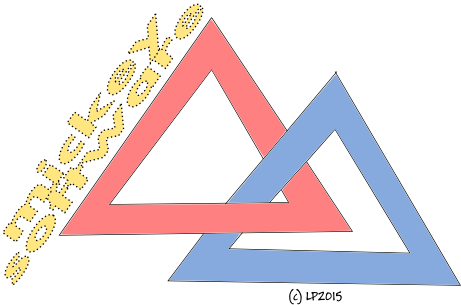+MICKEYsoftware presents

using System;
using System.Web.UI.WebControls;

{
protected void LoadL(object sender, EventArgs e)
{
string[] sou={"w","b","c","d","f","g","h","j","k","l","m","n","p","q","r","s","t","v","x","z"};
string[] samo={"a","e","i","o","u"};
Random rnd=new Random(DateTime.Now.Millisecond);

(sender as Label).Text="";int l=rnd.Next(2);
int max=rnd.Next(4)+4;
for (int i=0;i<=max;i++)
{
int sex;
if (rnd.Next(2)==0) sex=rnd.Next(2); else sex=0;
if (l==0)
(sender as Label).Text+=samo[rnd.Next(5)];
else
for (int j=0;j<=sex;j++)
{
int ind=0;
while (ind==0)
ind=rnd.Next(20);
(sender as Label).Text+=sou[ind];
}
if (l==0) l=1; else l=0;
}
(sender as Label).Text+=" ";l=rnd.Next(2);
max=rnd.Next(2);
for (int i=0;i<=max;i++)
{
if (max==0) l=0;
int sex;
if (rnd.Next(2)==0) sex=rnd.Next(2); else sex=0;
if (l==0)
(sender as Label).Text+=samo[rnd.Next(5)];
else
for (int j=0;j<=sex;j++)
{
int ind=0;
while (ind==0)
ind=rnd.Next(20);
(sender as Label).Text+=sou[ind];
}
if (l==0) l=1; else l=0;
}
(sender as Label).Text+=" ";l=rnd.Next(2);
max=rnd.Next(4)+4;
for (int i=0;i<=max;i++)
{
int sex;
if (rnd.Next(2)==0) sex=rnd.Next(2); else sex=0;
if (l==0)
(sender as Label).Text+=samo[rnd.Next(5)];
else
for (int j=0;j<=sex;j++)
{
int ind=0;
while (ind==0)
ind=rnd.Next(20);
(sender as Label).Text+=sou[ind];
}
if (l==0) l=1; else l=0;
}
}

}